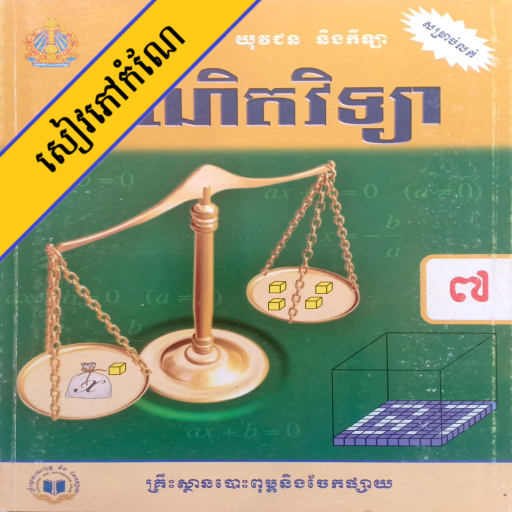# កំណែគណិតវិទ្យា ថ្នាក់ទី៧

4
|
70,243 Installs
|
|
Free

## កំណែគណិតវិទ្យា ថ្នាក់ទី៧ Description

Article list
Lesson 1: Integer 1
Lesson 2: Divorce and multiplication
Lesson 3: The number of Latin numerals
Lesson 4: Fraction
Lesson 5: Frequency
Lesson 6: Percentage
Lesson 7: Remarkable prizes
Lesson 8: Dexterity
Lesson 9: The first millimeter is one
Lesson 20: Inequality
Lesson 11: Ratio and Ratio
Lesson 12: The first notation of the geometry
Lesson 13: Angle
Lesson 14: The line breaks the corner
Lesson 15: Two-dimensional geometry
Lesson 16: Perimeter and polygon area
Lesson 17: Circles
Lesson 18: The volume and the surface of the solids
Lesson 19: Implications
Lesson 20: Probability
Lesson 21: Graph paper
Lesson 22: Plate